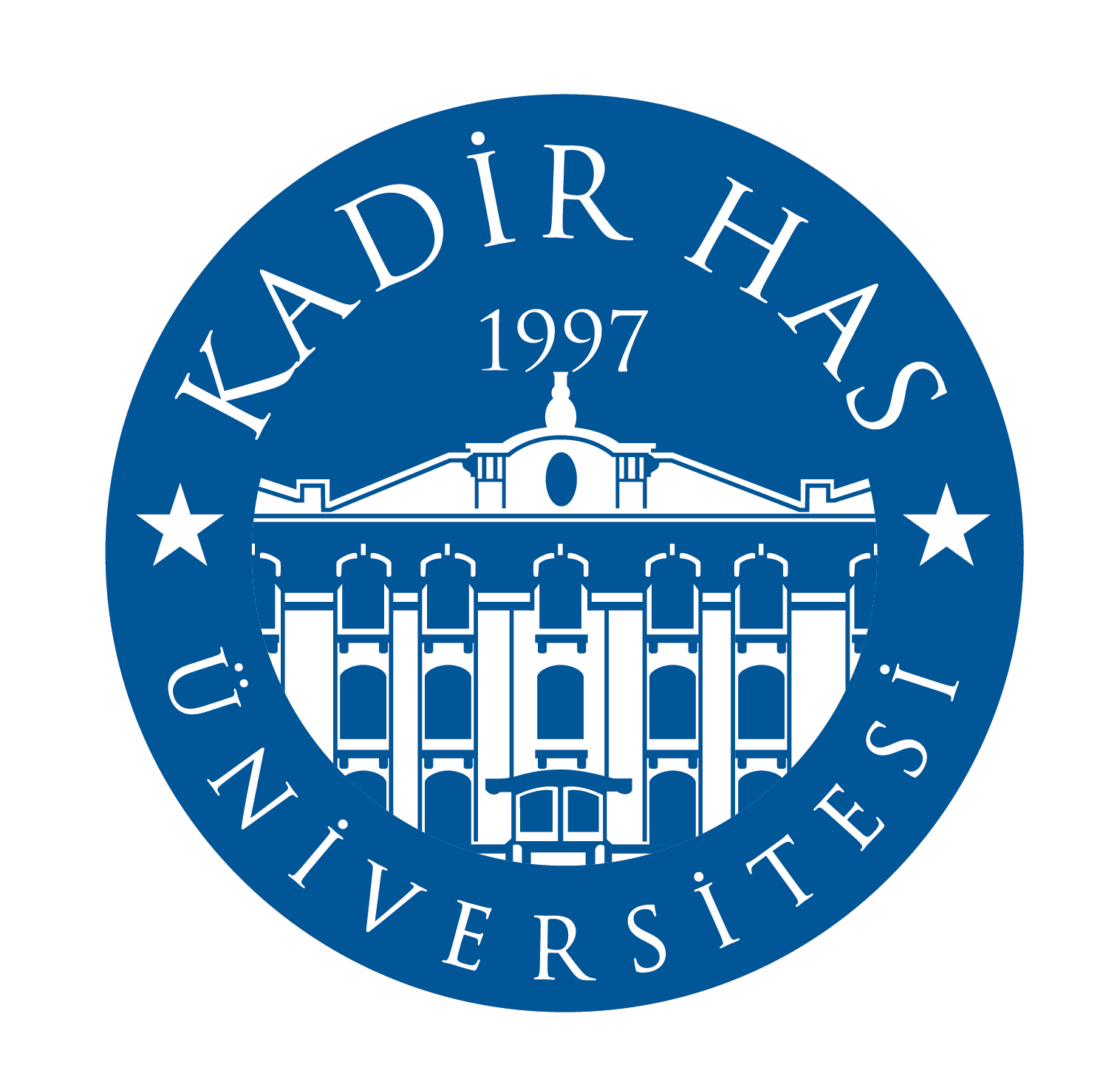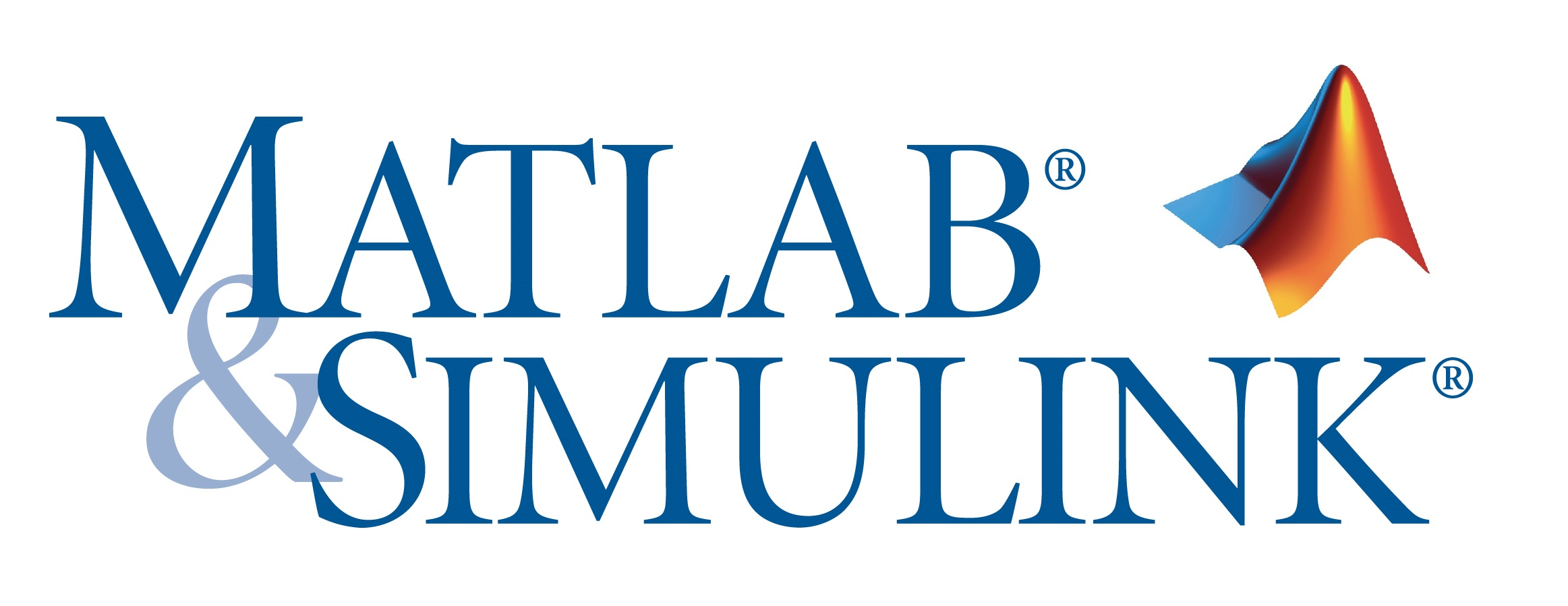# MATLAB for Kadir Has University

Kadir Has University has a Total Academic Headcount (TAH) license for MATLAB, Simulink, and add-on products. Faculty, researchers, and students may use these products for teaching, research, and learning. The license allows individuals to install the products on university-owned equipment, as well as personally owned computers.

MATLAB, the language of technical computing, is a programming environment for algorithm development, data analysis, visualization, and numeric computation. Simulink is a graphical environment for simulation and Model-Based Design of multidomain dynamic and embedded systems. MathWorks produces over 50 additional products for specialized tasks such as data analysis and image processing.### Products Available on Campus

• MATLAB
• Antenna Toolbox
• Bioinformatics Toolbox
• Communications System Toolbox
• Computer Vision System Toolbox
• Control System Toolbox
• Curve Fitting Toolbox
• DSP System Toolbox
• Data Acquisition Toolbox
• Database Toolbox
• Datafeed Toolbox
• Econometrics Toolbox
• Embedded Coder
• Filter Design HDL Coder
• Financial Instruments Toolbox
• Financial Toolbox
• Fixed-Point Designer
• Fuzzy Logic Toolbox
• Global Optimization Toolbox
• Image Processing Toolbox
• Instrument Control Toolbox
• MATLAB Coder
• MATLAB Compiler
• MATLAB Report Generator
• Mapping Toolbox
• Model Predictive Control Toolbox
• Neural Network Toolbox
• Optimization Toolbox
• Parallel Computing Toolbox
• Partial Differential Equation Toolbox
• RF Blockset
• RF Toolbox
• Robotics System Toolbox
• Robust Control Toolbox
• Signal Processing Toolbox
• Simscape
• Simscape Multibody
• Simscape Power Systems
• Stateflow
• Statistics and Machine Learning Toolbox
• Symbolic Math Toolbox
• System Identification Toolbox
• Wavelet Toolbox